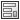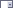Using Input Formula Aliases

When you use an alias in an input formula, the variable value selected in a control is evaluated to a different value in the input formula field.

The format of an alias in an input formula is as follows:

%VARIABLENAME(VALUE1=ALIAS1, VALUE2=ALIAS2)%

Where VALUE is the entered value in variable VARIABLENAME and ALIAS is the evaluated value.

When you use aliases in an input control, you create a single list control that contains a set of text strings defined in the Free text property. Then you create an edit box control and add an input formula that maps to the list control variable and the text strings.

This example describes how to create an input formula that allows the user to select a component from a list and view its price in an edit box.

To use an alias in an input formula:

1. Double-clickCards.
2. In the Card Editor, create a new card.
3. Add a Combobox Droplistcontrol.
4. In the Droplist properties pane:,
1. Under Value, click Variables.
2. Create a variable named Component.
3. Link the control to the variable.
4. Under Items, select Free text and type a list of components.
For example:
long_shaft
short_shaft
5. Add an edit box control.
6. In the Edit box properties pane:
1. Under Value, click Variables.
2. Create a variable named ComponentPrice.
3. Link the control to the variable ComponentPrice.
4. Beside Input formula, click Browse (...).
5. In the Input formula dialog box, type the input formula in the following format:
%Component(1st_component=1st_price, 2nd_component=2nd_price)%
For example:
%Component(long_shaft=$300, short_shaft=$200)%

7. Save the card.
When using the data card, if the user selects a component in the drop-down list, the edit box displays the alias associated with that component by the input formula.